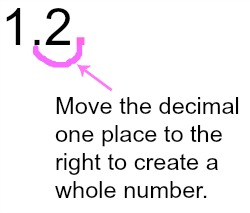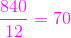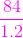# How to Divide a Whole Number by a Decimal

Instructor: Laura Pennington

Laura received her Master's degree in Pure Mathematics from Michigan State University. She has 15 years of experience teaching collegiate mathematics at various institutions.

This lesson will explain the extra steps we need to take to divide a whole number by a decimal. We will also go over why those extra steps work and we will look at some examples of carrying out this process.

## Steps to Solve

Most of us know how to divide a whole number by a whole number. There are many different algorithms that can be used to see how many times a divisor fits into a dividend when both the numbers are whole, but what do we do if the divisor is a decimal. In other words, how do we divide a whole number by a decimal? This is what we are going to explore and learn how to do.

Thankfully, to divide a whole number by a decimal, we just add a couple of steps to the process of dividing whole numbers, and these steps are not very difficult. They are as follows.

1. Count how many places we need to move the decimal to the right in the divisor to create a whole number.
2. Move the decimal this many places to the right in both the divisor and the dividend. This will require adding zeros to the end of the dividend.
3. You now have a division problem of two whole numbers and you can carry out the division using the algorithm that you are most comfortable with.

For example, suppose we wanted to divide the whole number 84 by the decimal 1.2. We would first count how many places we need to move the decimal to the right in 1.2 to get a whole number. This would be one place because if we move the decimal one place to the right, we get the whole number 12.The next step is to move the decimal one place to the right in both 84 and 1.2 to get 840 and 12. We now have 840 divided by 12, which is a division problem of whole numbers. We can carry out this division using the algorithm of our choice to get 70.## Solution

The steps we take to divide a whole number by a decimal are as follows.

1. Count how many places we need to move the decimal to the right in the divisor to create a whole number.
2. Move the decimal this many places to the right in both the divisor and the dividend. This will require adding zeros to the end of the dividend.
3. You now have a division problem of two whole numbers and you can carry out the division using the algorithm that you are most comfortable with.

## Why Does This Work?

This may leave you wondering why we can just move the decimal in this way. Wouldn't that change the problem? Thankfully, no, and we're going to see why. Let's look again at our example of 84 divided by 1.2 to illustrate why this process works.

In our example, we are dividing the whole number 84 by the decimal number 1.2. This can be illustrated in the following image.When we look at the decimal problem as a fraction 84 / 1.2 as shown in the image, we know that we can multiply both the numerator and denominator by the same number without changing the value of the fraction.

We saw that the first step is to count the number of places we need to move the decimal to the right in 1.2 to create a whole number, which is one. The next step is to move the decimal this many places to the right in both 84 and 1.2 to get 840 and 12.

Counting the number of decimal places we need to move to the right in 1.2 to get a whole number corresponds to finding what power of 10 we need to multiply 1.2 by in order to get a whole number. We said one place, and 1.2 x 10 1 = 1.2 x 10 = 12.

To unlock this lesson you must be a Study.com Member.

### Register to view this lesson

Are you a student or a teacher?

#### See for yourself why 30 million people use Study.com

##### Become a Study.com member and start learning now.
Back
What teachers are saying about Study.com

### Earning College Credit

Did you know… We have over 200 college courses that prepare you to earn credit by exam that is accepted by over 1,500 colleges and universities. You can test out of the first two years of college and save thousands off your degree. Anyone can earn credit-by-exam regardless of age or education level.